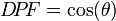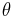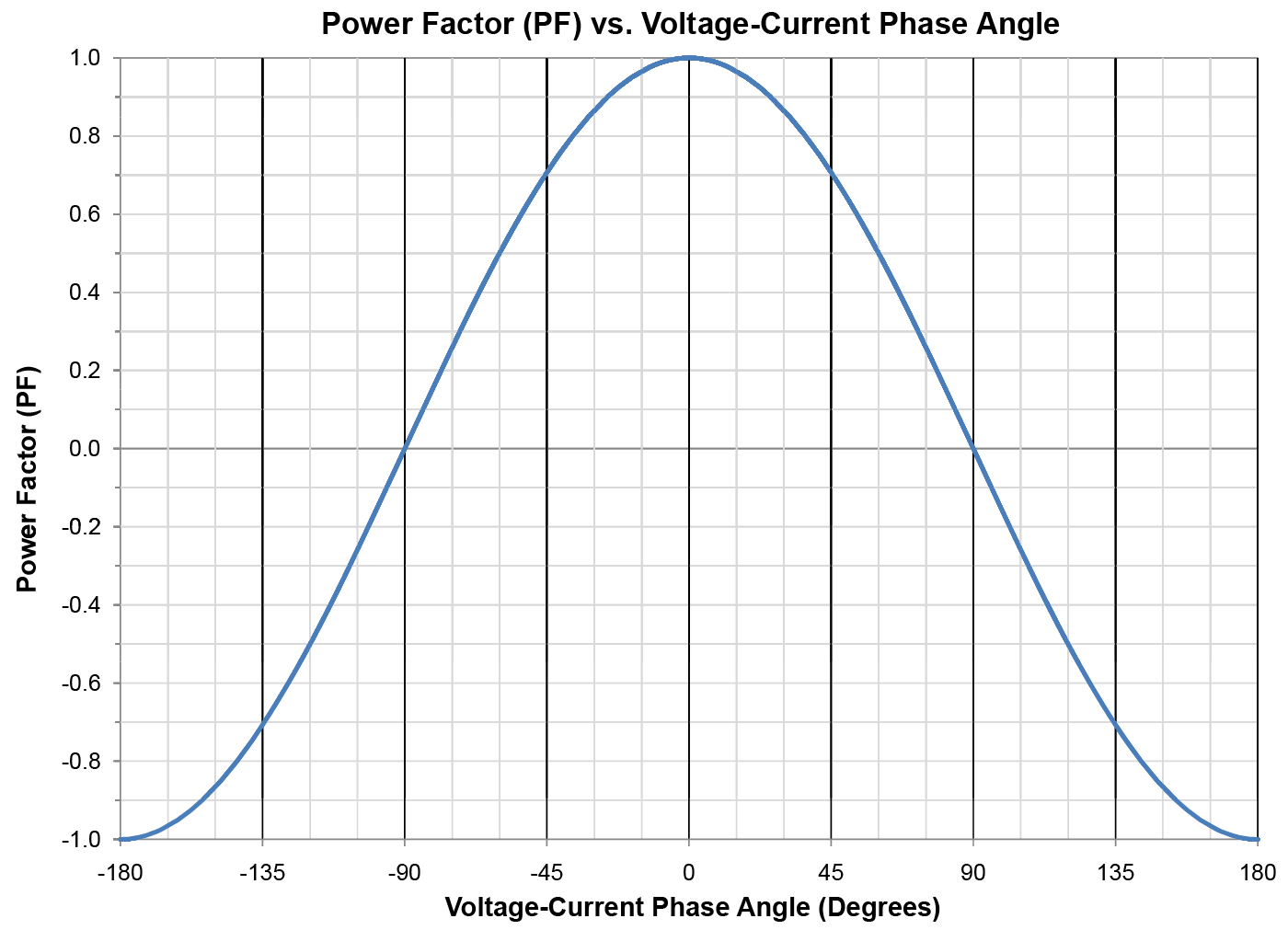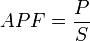The displacement power factor is the power factor due to the phase shift between voltage and current at the fundamental line frequency. For sinusoidal (non-distorted) currents, the displacement power factor is the same as the apparent power factor.$D!P!F = cos(theta)$ Where$theta$is the phase angle between the fundamental voltage and current. Inductive loads cause current to lag behind voltage, while capacitive loads cause current to lead voltage.The apparent power factor (APF) is the ratio of real power to apparent power, including harmonics.$APF = frac{P}{S}$ Where is the real power and is the apparent power. This includes all harmonics. Harmonic currents decrease the power factor, even when there is no phase angle between voltage and current.